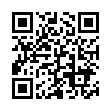# Basic Math 2013 F2 .pdf

### File information

Original filename: Basic Math - 2013 - F2.pdf
Author: Brian

This PDF 1.5 document has been generated by MicrosoftÂ® Word 2010, and has been sent on pdf-archive.com on 07/09/2017 at 07:48, from IP address 156.157.x.x. The current document download page has been viewed 457 times.
File size: 249 KB (8 pages).
Privacy: public file

Basic Math - 2013 - F2.pdf (PDF, 249 KB)

### Document preview

Candidate’s No. ……………………………………
THE UNITED REPUBLIC OF TANZANIA
MINISTRY OF EDUCATION AND VOCATIONAL TRAINING
FORM TWO SECONDARY EDUCATION EXAMINATION, 2013
0041

BASIC MATHEMATICS

TIME: 2½ HOURS
INSTRUCTIONS
1.
2.
3.
4.
5.
6.

This paper consists of sections A and B.
Answer ALL questions showing clearly all the working and answers in the
spaces provided.
ALL writing must be in blue or black ink EXCEPT drawings which must be in
pencil.
Write your examination number on the top right corner of every page.
Mathematical tables, geometrical instruments and graph papers may be used
where necessary.
Cellphones and calculators are not allowed in the examination room.

QUESTION
NUMBER
1
2
3
4
5
6
7
8
9
10
11
12
13

SCORE

FOR EXAMINER’S USE ONLY
INITIALS OF
QUESTION
EXAMINER
NUMBER
14
15
16
17
18
19
20
21
22
23
24
25

SCORE

TOTAL

This paper consists of 8 printed pages.

1

This and other free resources can be found at http://maktaba.tetea.org

INITIALS OF
EXAMINER

Candidate’s No. ……………………………………
SECTION A (60 MARKS)
1. The numbers 2, 3, 5 and Y have an average equal to 4. What is the number
represented by the letter Y?

2. Which numbers are equal to their squares?

5 3

3. Simplify the expression (3)

4. The difference in the measure of two supplementary angles is 102º. Find the two
angles.

5. In the year 2012, the population of the world was 6,760,000,000. Write this
population number in a scientific notation.

6. If N is a number such that when multiplied by 0.75 gives 1, find the value of N.

2

This and other free resources can be found at http://maktaba.tetea.org

Candidate’s No. ……………………………………
7. What percent of 1 hour is 15 minutes?

8. Write the fraction

31
8

as a mixed number.

9. What is 𝑥 if 𝑥 + 2𝑦 = 16 and 𝑦 = 3?

10. If 𝑥 2 − 𝑦 2 = 10 and 𝑥 + 𝑦 = 2, find the value of 𝑥 − 𝑦

11. Rationalize the denominator of the expression

2
3−√5

12. Simplify the algebraic expression −2(𝑥 − 3) + 4(−2𝑥 + 8)

3

This and other free resources can be found at http://maktaba.tetea.org

Candidate’s No. ……………………………………
13. Write the number 2373695 in words

14. Write 2 log 3 𝑥 + log 3 5 as a single logarithmic expression

15. Convert the expression 4 × 10−2 into decimal number.

16. If the area of a square tomato garden is 361 m2, find its perimeter.

17. What is the denominator when

5
6

is multiplied by

7
8

?

18. Without using mathematical tables simplify the expression √9 sin(162° − 72°)

4

This and other free resources can be found at http://maktaba.tetea.org

Candidate’s No. ……………………………………
19. Find the Least Common Multiple of 24, 36 and 48.

20. In a class of 75 form two students, 50 like Physics and 10 like Physics and Chemistry.
Apply the general formula to find the number of students who like Chemistry.

SECTION B (40 MARKS)
1

21. In a bag containing small balls, 4 are green,
26 are white. How many balls are blue?

5

1
8

are blue,

1
12

are yellow and the remaining

This and other free resources can be found at http://maktaba.tetea.org

Candidate’s No. ……………………………………
22. Find the size of angle 𝑥 in the figure below.

C
94°

E
x

A

110°

41°
D

B

3
4

23. In a right angled triangle, tan 𝐴 = . Find sin 𝐴 and cos 𝐴.

6

This and other free resources can be found at http://maktaba.tetea.org

Candidate’s No. ……………………………………
24. Draw a Venn diagram to represent the relationship between the sets
𝐴 = {1, 2, 5, 6, 7, 9, 10} and 𝐵 = {1, 3, 4, 5, 6, 8, 10}

25. The following pie chart shows a survey of the number of cars, buses and motorcycles
which pass a particular junction. The survey shows that there were 150 buses which
were passing the junction.

MOTORCYLES

210°
30°
120°
BUSES

CARS
From the given information determine:

7

This and other free resources can be found at http://maktaba.tetea.org

Candidate’s No. ……………………………………
(i)

The fraction of motorcycles.

(ii)

The percentage of cars which were passing the junction.

(iii)

The total number of the vehicles (motorcycles, cars and buses).

8

This and other free resources can be found at http://maktaba.tetea.org

#### HTML Code

Copy the following HTML code to share your document on a Website or Blog

#### QR Code### Related keywords